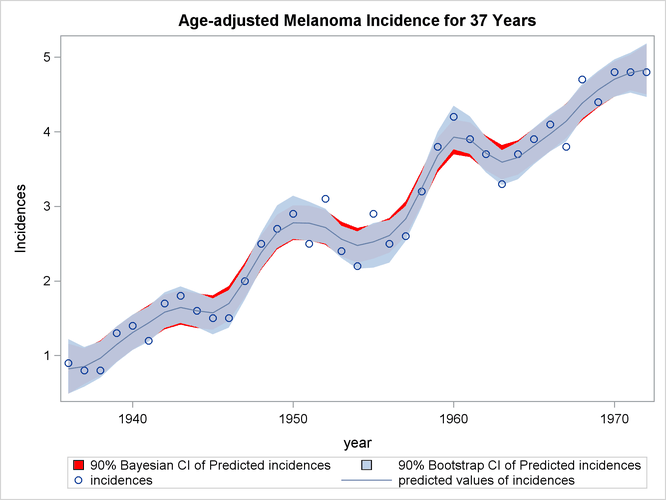# The TPSPLINE Procedure

### Example 96.5 Computing a Bootstrap Confidence Interval

This example illustrates how you can construct a bootstrap confidence interval by using the multiple responses feature in PROC TPSPLINE.

Numerous epidemiological observations have indicated that exposure to solar radiation is an important factor in the etiology of melanoma. The following data present age-adjusted melanoma incidences for 37 years from the Connecticut Tumor Registry (Houghton, Flannery, and Viola, 1980). The data are analyzed by Ramsay and Silverman (1997).

data melanoma;
input  year incidences @@;
datalines;
1936    0.9   1937   0.8  1938   0.8  1939   1.3
1940    1.4   1941   1.2  1942   1.7  1943   1.8
1944    1.6   1945   1.5  1946   1.5  1947   2.0
1948    2.5   1949   2.7  1950   2.9  1951   2.5
1952    3.1   1953   2.4  1954   2.2  1955   2.9
1956    2.5   1957   2.6  1958   3.2  1959   3.8
1960    4.2   1961   3.9  1962   3.7  1963   3.3
1964    3.7   1965   3.9  1966   4.1  1967   3.8
1968    4.7   1969   4.4  1970   4.8  1971   4.8
1972    4.8
;


The variable incidences records the number of melanoma cases per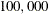people for the years 1936 to 1972. The following model fits the data and requests a 90% Bayesian confidence interval along with the estimate:

ods graphics on;
proc tpspline data=melanoma plots(only)=(criterionplot fitplot(clm));
model incidences = (year) /alpha = 0.1;
output out = result pred uclm lclm;
run;


The output is displayed in Output 96.5.1

Output 96.5.1: Output from PROC TPSPLINE for the MELANOMA Data

 Comparison of Two Estimates with and without the D= Option

The TPSPLINE Procedure
Dependent Variable: incidences

Summary of Input Data Set
Number of Non-Missing Observations 37
Number of Missing Observations 0
Unique Smoothing Design Points 37

Summary of Final Model
Number of Regression Variables 0
Number of Smoothing Variables 1
Order of Derivative in the Penalty 2
Dimension of Polynomial Space 2

Summary Statistics of Final Estimation
log10(n*Lambda) -0.0607
Smoothing Penalty 0.5171
Residual SS 1.2243
Tr(I-A) 22.5852
Model DF 14.4148
Standard Deviation 0.2328
GCV 0.0888

The estimated curve is displayed with 90% confidence interval bands in Output 96.5.2. The number of melanoma incidences exhibits a periodic pattern and increases over the years. The periodic pattern is related to sunspot activity and the accompanying fluctuations in solar radiation.

Output 96.5.2: PROC TPSPLINE Estimate and 90% Confidence Interval of Data Set MELANOMA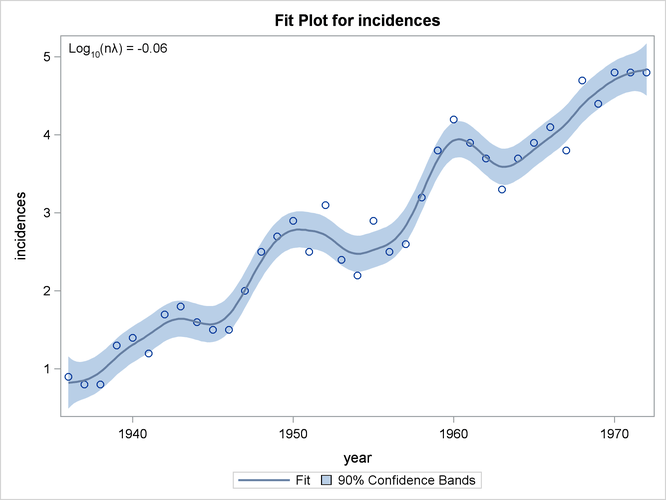Wang and Wahba (1995) compare several bootstrap confidence intervals to Bayesian confidence intervals for smoothing splines. Both bootstrap and Bayesian confidence intervals are across-the-curve intervals, not pointwise intervals. They concluded that bootstrap confidence intervals work as well as Bayesian intervals concerning average coverage probability. Additionally, bootstrap confidence intervals appear to be better for small sample sizes. Based on their simulation, the percentile-t interval bootstrap interval performs better than the other types of bootstrap intervals.

Suppose that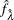and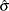are the estimates of f and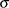from the data. Assume thatis the true f, and generate the bootstrap sample as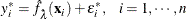where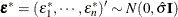. Denote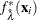as the random variable of the bootstrap estimate at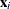. Repeat this process K times, so that at each point, you have K bootstrap estimates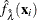or K realizations of. For each fixed, consider the statistic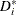, which is similar to the Student’s t statistic,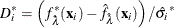where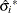is the estimate ofbased on the ith bootstrap sample.

Suppose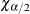and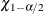are the lower and upper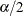points, respectively, of the empirical distribution of. The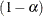100% bootstrap confidence interval is defined as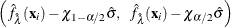Bootstrap confidence intervals are easy to interpret and can be used with any distribution. However, because they require K model fits, their construction is computationally intensive.

The feature of multiple dependent variables in PROC TPSPLINE enables you to fit multiple models with the same independent variables. The procedure calculates the matrix decomposition part of the calculations only once, regardless of the number of dependent variables in the model. These calculations are responsible for most of the computing time used by the TPSPLINE procedure. This feature is particularly useful when you need to generate a bootstrap confidence interval.

To construct a bootstrap confidence interval, perform the following tasks:

• Fit the data by using PROC TPSPLINE and obtain estimatesand.

• Generate K bootstrap samples based onand.

• Fit the K bootstrap samples with the TPSPLINE procedure to obtain estimates of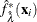and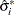.

• Computeand the valuesand.

The following statements illustrate this process:

proc tpspline data=melanoma plots(only)=fitplot(clm);
model incidences = (year) /alpha = 0.1;
output out=result pred uclm lclm;
run;


The output from the initial PROC TPSPLINE analysis is displayed in Output 96.5.3. The data set result contains the predicted values and confidence limits from the analysis.

Output 96.5.3: Output from PROC TPSPLINE for the MELANOMA Data

 Comparison of Two Estimates with and without the D= Option

The TPSPLINE Procedure
Dependent Variable: incidences

Summary of Input Data Set
Number of Non-Missing Observations 37
Number of Missing Observations 0
Unique Smoothing Design Points 37

Summary of Final Model
Number of Regression Variables 0
Number of Smoothing Variables 1
Order of Derivative in the Penalty 2
Dimension of Polynomial Space 2

Summary Statistics of Final Estimation
log10(n*Lambda) -0.0607
Smoothing Penalty 0.5171
Residual SS 1.2243
Tr(I-A) 22.5852
Model DF 14.4148
Standard Deviation 0.2328
GCV 0.0888

The following statements illustrate how you can obtain a bootstrap confidence interval for the Melanoma data set. The following statements create the data set bootstrap. The observations are created with information from the preceding PROC TPSPLINE execution; as displayed in Output 96.5.3,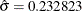. The values ofare stored in the data set result in the variable P_incidence.

data bootstrap;
set result;
array y{1070} y1-y1070;
do i=1 to 1070;
y{i} = p_incidences + 0.232823*rannor(123456789);
end;
keep y1-y1070 p_incidences year;
run;

ods listing close;
proc tpspline data=bootstrap plots=none;
ods output FitStatistics=FitResult;
id p_incidences;
model y1-y1070 = (year);
output out=result2;
run;
ods listing;


The DATA step generates 1,070 bootstrap samples based on the previous estimate from PROC TPSPLINE. For this data set, some of the bootstrap samples result ins (selected by the GCV function) that cause problematic behavior. Thus, an additional 70 bootstrap samples are generated.

The ODS listing destination is closed before PROC TPSPLINE is invoked. The PLOTS=NONE option suppresses all graphical output. The model fits all the y1y1070 variables as dependent variables, and the models are fit for all bootstrap samples simultaneously. The output data set result2 contains the variables year, y1y1070, p_y1p_y1070, and p_incidences.

The ODS OUTPUT statement writes the FitStatistics table to the data set FitResult. The data set FitResult contains the two variables Parameter and Value. The FitResult data set is used in subsequent calculations for.

In the data set FitResult, there are 63 estimates with a standard deviation of zero, suggesting that the estimates provide perfect fits of the data and are caused by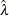s that are approximately equal to zero. For small sample sizes, there is a positive probability that thechosen by the GCV function will be zero (Wang and Wahba, 1995).

In the following steps, these cases are removed from the bootstrap samples as bad samples: they represent failure of the GCV function.

The following SAS statements manipulate the data set FitResult, retaining the standard deviations for all bootstrap samples and merging FitResult with the data set result2, which contains the estimates for bootstrap samples. In the final data set boot, thestatistics are calculated.

data FitResult;
set FitResult;
if Parameter="Standard Deviation";
keep Value;
run;

proc transpose data=FitResult out=sd prefix=sd;

data result2;
if _N_ = 1 then set sd;
set result2;
run;

data boot;
set result2;
array y{1070}  p_y1-p_y1070;
array sd{1070} sd1-sd1070;
do i=1 to 1070;
if sd{i} > 0 then do;
d = (y{i} - P_incidences)/sd{i};
obs = _N_;
output;
end;
end;
keep d obs P_incidences year;
run;


The following SAS statements retain the first 1,000 bootstrap samples and calculate the valuesandwith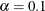.

proc sort data=boot;
by obs;
run;

data boot;
set boot;
by obs;
retain n;

if first.obs then n=1;
else n=n+1;
if n > 1000 then delete;
run;

proc sort data=boot;
by obs d;
run;

data chi1 chi2 ;
set boot;
if (_N_ = (obs-1)*1000+50)  then output chi1;
if (_N_ = (obs-1)*1000+950) then output chi2;
run;

proc sort data=result;
by year;
run;

proc sort data=chi1;
by year;
run;

proc sort data=chi2;
by year;
run;

data result;
merge result
chi1(rename=(d=chi05))
chi2(rename=(d=chi95));
keep year incidences P_incidences lower upper
LCLM_incidences UCLM_incidences;

lower = -chi95*0.232823 + P_incidences;
upper = -chi05*0.232823 + P_incidences;

label  lower="Lower 90% CL (Bootstrap)"
upper="Upper 90% CL (Bootstrap)"
lclm_incidences="Lower 90% CL (Bayesian)"
uclm_incidences="Upper 90% CL (Bayesian)";
run;


The data set result contains the variables year and incidences, the PROC TPSPLINE estimate P_incidences, and the 90% Bayesian and 90% bootstrap confidence intervals.

The following statements produce Output 96.5.4:

proc sgplot data=result;
title "Age-adjusted Melanoma Incidence for 37 Years";

xaxis label="year";
yaxis label="Incidences";

band x=year lower=lclm_incidences upper=uclm_incidences/name="bayesian"
legendlabel="90% Bayesian CI of Predicted incidences"
fillattrs=(color=red);
band x=year lower=lower upper=upper/name="bootstrap"
legendlabel="90% Bootstrap CI of Predicted incidences"
transparency=0.05;
scatter x=year y=incidences/name="obs" legendlabel="incidences";
series x=year y=p_incidences/name="pred"
legendlabel="predicted values of incidences"
lineattrs=graphfit(thickness=1px);

discretelegend "bayesian" "bootstrap" "obs" "pred";
run;

ods graphics off;


Output 96.5.4 displays the plot of the variable incidences, the predicted values, and the Bayesian and bootstrap confidence intervals.

The plot shows that the bootstrap confidence interval is similar to the Bayesian confidence interval. However, the Bayesian confidence interval is symmetric around the estimates, while the bootstrap confidence interval is not.

Output 96.5.4: Comparison of Bayesian and Bootstrap Confidence Interval for Data Set MELANOMA